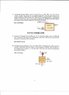# Mixing chamber with multiple inlets

I don't know what you're trying to say.Thanks for trying to help, but I don't think that was necessary.f

Hi I was wondering if someone could help me with this question. Question 4 uploaded in picture.
Ok, so far this is what I have found

Pipe 1:
m1=2kg/s
P1=1000kpa
T1=100 C
h1=334.82kJ/kg

Pipe 2:
sat-liquid
T2=60 C
P2= 1000kpa
h2=291.36KJ/kg

Outlet:
sat vapor
P3=1000kpa
V=20 m/s
h3=267.97 kJ/kg
v3= 0.0202 m^3/kg

and I know that the mass in = mass out
so m1 + m2 = mout
My problem is that I don't know what to do with the velocity because I do not have area to use formula Vout=VA

Also I believe to solve the problem the formula m1*h1 + m2*h2 = m3*h3
but I have tried a variation of this and it did not work.

#### Attachments

•thermo.jpg
16.1 KB · Views: 677
Enthalpy is conserved for each input, right. That's because dH = dQ + Vdp, dQ = 0, dp = 0. So you have m1h1 + m2h2 = m3h3. You also have m1 + m2 = m3. In other words, you've already stated all the equations you need.

Two equations, 2 unknowns (m2 ans m3). Outflow = m3*V.

I'm not claiming total knowledge here - does anyone see any flaws in this?

Enthalpy is conserved for each input, right. That's because dH = dQ + Vdp, dQ = 0, dp = 0. So you have m1h1 + m2h2 = m3h3. You also have m1 + m2 = m3. In other words, you've already stated all the equations you need.

Two equations, 2 unknowns (m2 ans m3). Outflow = m3*V.

I'm not claiming total knowledge here - does anyone see any flaws in this?

I have tied to use that method which would be
m1h1 + m2h2 = (m1 + m2)h3
but then m2 = -5.716 and this is wrong
I don't now how to relate the velocity into the equation. You said that outflow = m3*V right? what would outflow be? wouldn't the units be like kgm/s^2 or something like that?

My apologies. I did not think when I wrote that outflow = m3*V. Outflow should be just m3.

Something bothers me. Your enthalpy at the outlet is less than the enthalpy at inlet 2. That sounds weird. At 2 we have saturated liquid, and at 3 we have saturated vapor , so h3 must be > h2, yet you have h3 < h2. h3 looks OK since its temp. is 100 vs. 60 for the other two, so its h should be the highest of all.

I don't know what to do with V, if it's a red herring or needed. Must think more.

EDIT:

To conserve energy, looks like h3 should be reduced by ρV^2 where ρ = density at outlet.

BTW your insert is really hard to read, at least on my display.

Last edited:
Actually I believe the h3 value is correct because r-134a at 1000kpa the saturation temp is only 39.39 C and the sat vapor value in the table hg= 267.97. The numbers are much less intuitive because it is not water. Still confused by this velocity given. It feels like It is a necessary piece of info but without area of pipe or diameter I can't seem to do anything with it.

According to my table, saturated liquid enthalpy of r134a at 1 MPa is 105.29 kg/kJ, not 291.36. The table agrees with your T = 39.39C and sat. vapor h of 267.97.

http://energy.sdsu.edu/testhome/Test/solve/basics/tables/tablesPC/pSatR134a.html

I don't see how inlet 2 can have T = 60C and p = 1 Mpa. There's something fishy about that. As you can see from the table, and as I believed a priori, there is one and only one temperature associated with saturated r134a at 1 MPa, and that is 39.39C. I wonder if there is something tricky about the statement "after the valve", though I sure can't see it.

Oh my bad you are very correct. I read the table wrong. I believe the pressure at inlet 2 is so high due to the valve.

hmm. I'm not sure. Like there shouldn't be. min to valve should = mout

Sorry is this better?

Much! Thanks.

Ok I got it. So h3 will be 267.97 because it is sat vapor at 1Mpa and h2 will be 137.42 which is the sat-liquid value for 60 C at a pressure of 1681.3 Kpa. And because the hin = hout of a valve this can be used. Plug all this in the the formula we talked about and a got the right answer. Thanks for your help and for your time.

You absolutely got it. I was just writing to point out that the valve made it a throttling process with h conserved. Good going!

PS so V was never used?

You absolutely got it. I was just writing to point out that the valve made it a throttling process with h conserved. Good going!

PS so V was never used?

Nope. I guess it was to throw you off as well as the pressure at p2 after the valve was never used. I hate extra info that that. Thanks again